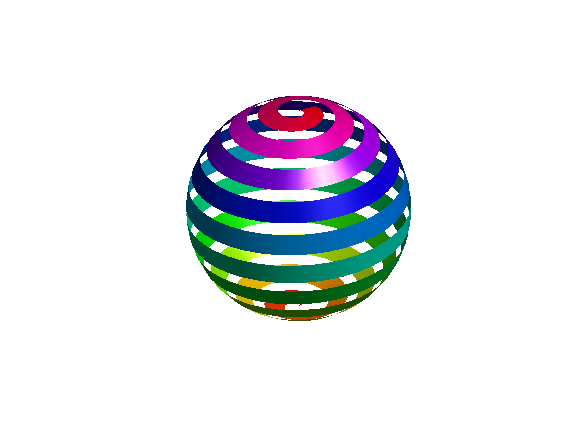• /
• # Spiraled Sphere

on 29 Oct 2022
• 4
• 36
• 1
• 0
• 179
% A sphere made of a spiral ribbon
%n=40;% Resolution of the sphere
%S=10;% Number of spirals
w=.06;% (1/S)*t; % Thickness of the ribbon as a factor of other vars
% Theta goes around S times, with n verts per round.
l=@linspace;
T=l(0,20,400);% (0,S*2,n*S)
T=[T;T];% top and bottom of ribbon
% Phi slowly goes from -.5 to .5 (top+bottom of sphere) with same # verts
% as theta.
P=l(-.5+w,.5,400);
P=[P;P-w];% top and bottom of ribbon, thickness of w.
% Make the surface coordinates
c=@cospi;
s=@sinpi;
X=c(P).*c(T);
Y=c(P).*s(T);
Z=s(P);
% Draw
surf(X,Y,Z,T);